# Aluminium chloride (AlCl3) lewis dot structure, molecular geometry, polar or non-polar, hybridization

Home  > Chemistry Article > AlCl3 lewis structure and its molecular geometry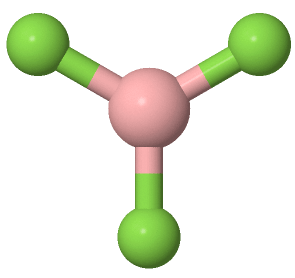Aluminium chloride is composed of aluminium and chloride also known as aluminium trichloride or aluminium (III) chloride having the chemical formula AlCl3. It appears as white to gray powder with a pungent odor. In solution forms, it appears as a straw-colored liquid. It is mostly used in the production of aluminium metals.

In this tutorial, we will study Aluminium chloride (AlCl3) lewis structure, molecular geometry, hybridization, polarity, bond angle, etc.

Aluminium chloride is corrosive to tissue and toxic by ingestion. It is powerful lewis acid and capable of reversible changing from polymer to monomer at mild temperature. It sometimes appears yellowish in color due to the presence of contaminants.

Properties of Aluminium chloride

• It has a molar mass of 133.341 g/mol.
• It appears as white or pale yellow solid.
• It has a melting point of 180 °C.
• It has a low melting and boiling point.
• It is non-flammable and powerful lewis acid.
• It has a coordinate geometry of octahedral in the solid phase and tetrahedral in the liquid phase.
 Name of Molecule Aluminium chloride Chemical formula AlCl3 Molecular geometry of AlCl3 Trigonal planar Electron geometry of AlCl3 Trigonal planar Hybridization Sp2 Nature Non-polar molecule Total Valence electron for AlCl3 24
Page Contents

## How to draw lewis structure for AlCl3?

AlCl3 lewis structure contains three bonded pairs and a total of 9 lone pairs. The central atom of the AlCl3 lewis structure is exceptional to the octet rule as it holds stability with only 6 electrons.

In this tutorial, we will draw the lewis structure of Aluminum chloride with simple steps and all possible explanations.

## Follow some steps for drawing the lewis dot structure of AlCl3

1. Count total valence electron in AlCl3

Like every other lewis diagram procedure, we have to first count the total number of valence electrons available for AlCl3. One of the easy ways to find out the valence electron of an atom is to remember its periodic group.

As aluminium belongs to the periodic group of 13th and chlorine is present in the 17th periodic group. Hence, the valence electron of aluminium is three and for chlorine, it is seven.

⇒ Total number of the valence electrons in Aluminium = 3

⇒ Total number of the valence electrons in Chlorine = 7

∴ Total number of valence electrons available for drawing the lewis structure of AlCl3 = 3 + 7(3) = 24 valence electrons         [∴AlCl3 molecule has one aluminium and three chlorine atoms]2. Find the least electronegative atom and placed it at center

Electronegativity increase from left to right in the periodic table. Aluminium is situated in the 13th periodic group and chlorine in the 17th group. Hence, aluminium is on the left side in the periodic group and obviously, chlorine is at the right to the aluminium atom.

Therefore, the aluminium atom(1.61) is less electronegative than the chlorine atom(3.16). So, just put the aluminium at the central position and chlorine atoms spaced evenly around it.3. Connect outer atoms to central atom with a single bond

Now join each outer atom(chlorine) to the central atom(aluminium) with a single bond.Count the number of valence electrons we used in the above structure. There are three single bonds used in the above figure for connecting each chlorine atom to the aluminium central atom. And one single bond composed of two electrons.

Hence, three single bonds mean (3 × 2) = 6 valence electrons are used in the above structure from a total of 24 valence electrons available for AlCl3.

∴ (24 – 6) = 18 valence electrons

So, we are left with 18 valence electrons more.

4. Place remaining valence electrons starting from outer atom first

In this step, we will complete the octet of outer atoms i.e. chlorine. Afterward, we will go for the central atom(aluminium).

Chlorine needs 8 electrons in the outermost shell to complete the octet. Hence, just put over the remaining valence electrons on chlorine atoms first.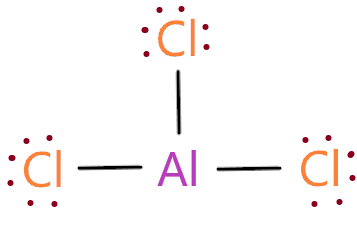As you see in the above figure, we put the 6 electrons on each chlorine atom as they have already 2 electrons from the attached single bond. So, each chlorine atom successfully completed the octet as all have 8 electrons in their outermost shell.

5. Complete central atom octet and make covalent bond if necessary

We already completed the octet of the outer atom, now we will look for the central atom(aluminium). It should be noted that we had a total of 24 valence electrons available for drawing the lewis structure of AlCl3.

But in the 4th step structure, we used all the 24 valence electrons(18 electrons represented as dots and three single bonds that contain 6 electrons).

So, we don’t have any extra valence electrons left. Aluminium central atom shares 6 valence electrons through the three single bonds attached to it.

It should be noted that Aluminium is exceptional to the octet rule as it can have 8 electrons or less than 8 electrons in the outermost shell to attain stability. Aluminum is an exception just like boron where it can be octet deficient.

Octet deficient molecules are the molecules that can attains the stability by having less than 8 electrons around the atoms. Some examples – Boron, beryllium, aluminium, hydrogen, lithium, helium

But boron and aluminium is two most common element that can fail to complete the octet as they attains stability having only 6 valence electrons.

Therefore, aluminium central atom in the AlCl3 lewis structure attains stability by just having 6 valence electrons around it.

Many of you have the question why can’t we convert the lone pair of chlorine atoms to make a double bond and provide the 8 electrons to the central atom aluminium.

To understand it let’s move to the next step.

6. Check the stability with the help of a formal charge concept

“As we know, the lesser the formal charge on atoms, the better is the stability of the lewis diagram.”

⇒ Formal charge = (valence electrons – lone pair electrons –  1/2 bonded pair electrons)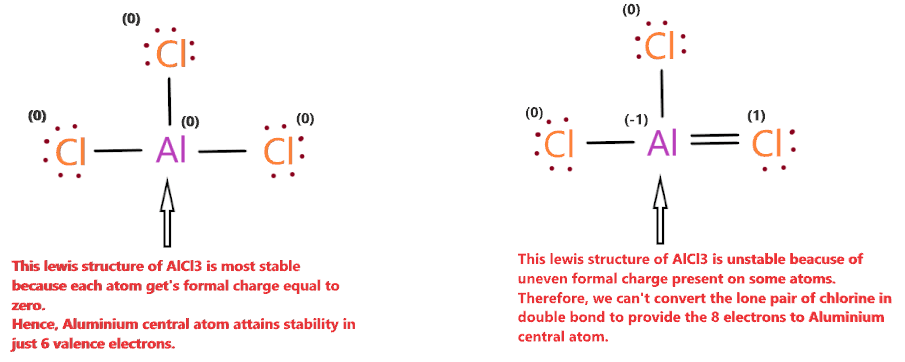As you see in the above figure, the left side structure of AlCl3 gets zero formal charges on each atom, thus, it is most stable. Whereas right side AlCl3 structure gets an uneven formal charge on some atoms, thus, it is unstable than the left side structure.

At last, if you are ever confused about exceptional to an octet in lewis diagram, make sure you should check the formal charges. A lewis diagram that gets formal charge zero or close to zero is most stable.

Therefore, Aluminium central atom in the AlCl3 lewis structure is attained stability by just having 6 valence electrons around it because of lesser formal charge on atoms.

## What is the molecular geometry of AlCl3?

The molecular geometry of AlCl3 is trigonal planar because the central atom aluminium is bonded with three chlorine atoms and it contains no lone pair, this means, distortion around the central position will not happen because of no lone pair.

Hence, the three bonded atoms(chlorine) are arranged like a triangle around the central atom(aluminium)

Also, as per VSEPR theory, three regions (3 bonded atoms) with no lone pair electrons on the central atom always forms the trigonal planar geometry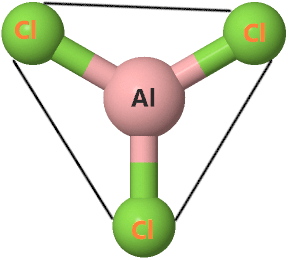Let’s find the molecular geometry of AlCl3 using the AXN method.

AXN notation for AlCl3 molecule:

• A represents the central atom, so, in the AlCl3 molecule, aluminium is the central atom. A = Aluminium
• X represents the bonded atoms to the central atom, so, the aluminium atom is bonded with three chlorine atoms. Therefore, X = 3
• N represent the lone pair on the central atom, as per the AlCl3 lewis structure, the central aluminium atom doesn’t have any lone pair. Hence, N = 0

So, the AXN generic formula for the AlCl3 molecule becomes AX3N0 or AX3.

The VSEPR theory said if the molecule has an AX3 formula then its molecular and electron geometry will be trigonal planar.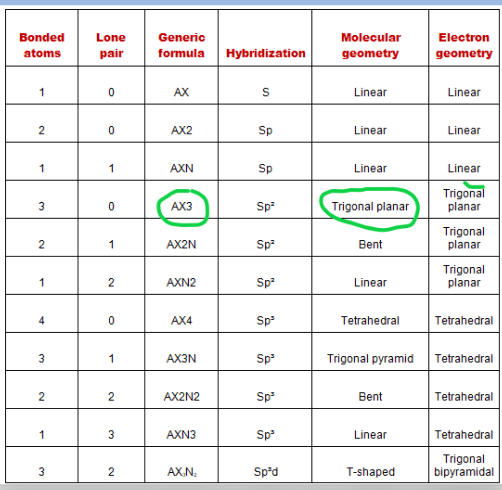Hence, the molecular shape for AlCl3 is trigonal planar and its electron geometry is also trigonal planar.## Hybridization of AlCl3

The hybridization of AlCl3 is Sp2 because the steric number of the aluminium central atoms is three.

“Three atoms hybridise with Al to undergo sp2 hybridisation to give a trigonal planar arrangement in AlCl3”

The calculation of steric number is done by adding a number of bonded atoms attached to the central atom and lone pair on the central atom.

In the case of the AlCl3 molecule, aluminium is the central atom that is attached to the three bonded atoms(chlorine) and it has no lone pairs.

Hence, (3 + 0) = 3 is the steric number of central atom aluminium in the AlCl3 molecule that gives Sp2 hybridization.

 Steric number Hybridization 1 S 2 Sp 3 Sp² 4 Sp³ 5 Sp³d 6 Sp³d²

## The bond angle of AlCl3

The three Al-Cl bonds are evenly arranged in the same plane. The bonds are spread equally around the plane, forming 120-degree bond angles.

## Aluminium chloride polarity: is AlCl3 polar or nonpolar?

So, Is AlCl3 polar or nonpolar? Well, it is obvious that AlCl3 is a nonpolar molecule because each Al-Cl bond is directed at the angle of 120° to each other in a plane, hence, canceling of dipole moment generated along these bonds is very easy.

Therefore, no dipole moment is generated in the AlCl3 molecule, hence, it is nonpolar in nature.

Also, the molecular geometry of AlCl3 is very symmetrical since no lone pair is present on the central atom that can cause distortion in a molecule, so, the charges are distributed uniformly all over the atoms.

Hence, the dipole generated in the AlCl3 molecule will easily cancel out each other leaving this molecule nonpolar in nature.

## FAQ

### Why is the molecule geometry of AlCl3 is trigonal planar and not trigonal pyramidal?

According to the VSEPR theory, any molecule that has three regions of electron density with no lone pair on the central atom always forms a trigonal planar geometry whereas a molecule that has three regions of electron density with the presence of lone pair on the central atom always forms a trigonal pyramidal geometry.

Since the AlCl3 molecule doesn’t contain any lone pair on the central atom and has a three region of electron density, hence, it forms a trigonal planar geometry instead of trigonal pyramidal geometry.

### Does the AlCl3 lewis structure violate the octet?

Yes, the Aluminium chloride lewis structure violates the octet because its central atom attains stability by having just 6 valence electrons in outermost shells instead of 8 electrons.

### Why Aluminium central atom of the AlCl3 lewis structure complete the octet in just 6 electrons?

This is because aluminium is the most common element that can fail to complete the octet as it attains stability having only 6 valence electrons. Aluminium is exceptional to the octet and it is also called octet deficient.

But in the case of the AlCl3 lewis diagram, the Aluminium central atom get’s a formal charge equal to zero when it has 6 electrons around. But when an aluminium central atom is distributed with 8 electrons it gets an uneven formal charge.

Hence, we have to choose the lewis diagram that has the least formal charge on each atom, Therefore, the aluminium central atom is provided with only 6 electrons instead of 8 for completing the octet shell.

## Summary

• The total valence electron is available for drawing the Aluminium chloride lewis structure is 24.
• The hybridization of the AlCl3 molecule is Sp2 since it has a steric number equal to 3 that will form an Sp2 hybrid.
• AlCl3 is a nonpolar molecule because its net dipole moment is zero and charges are uniformly distributed all over the atom.
• In the AlCl3 lewis structure, a total of 9 lone pairs are present but no lone pair on the central atom.
• The molecular geometry of AlCl3 is trigonal planar with each Al-Cl bond angeled 120° to each other and its electron geometry is also trigonal planar.
##### Subscribe to Blog via Email

Join 2 other subscribers

Share it...